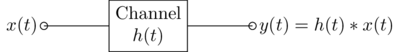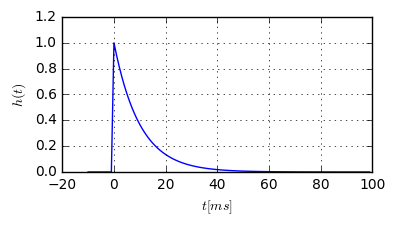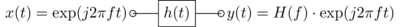# An intuitive explanation on how OFDM works¶

This article is about the fundamental principle of OFDM. Without going into the mathematical details, I'm going to explain, why the commonly used OFDM waveform is designed as it is. In a previous article, we have already shown a source code example of OFDM.

Let us start with the assumption that we face a static multipath channel (static means, the channel coefficients do not change over all times, i.e. it is time-invariant). Such a channel is a linear, time-invariant (LTI) system, and hence it is described solely by its impulse response $h(t)$. The output signal $y(t)$ of this system is the convolution of the input signal $x(t)$ with the impulse response $h(t)$:### Eigenfunctions of the time-invariant channel¶

The most important property of LTI systems is, that complex exponentials of any frequency are eigenfunctions of the system. Literally, if the input to an LTI system is a complex exponential, its output will be a complex exponential of the same frequency, but with a different amplitude. Let's verify this numerically (here we resort to real-valued functions since they are easier to draw). First, let's define some impulse response of our channel:

Fs = 1000 # Sampling frequency for discretizing the simulation
t_imp = np.arange(-10e-3, 100e-3, 1/Fs)
h = np.exp(-0.1*t_imp*1000) * (t_imp >= -0.00001) * (t_imp < 100e-3)
plt.plot(t_imp*1000, h);We have defined a causal impulse response, lasting 100ms. Now, let's send some harmonic functions through this system:def showEigenfunction(f):
t = np.arange(-1, 1, 1/Fs)
x = np.cos(2*np.pi*f*t)
h = np.exp(-np.arange(10)/2)

plt.subplot(121)
plt.plot(t*1000, x)

plt.subplot(122)
y = np.convolve(h, x)
plt.plot(t*1000, y)